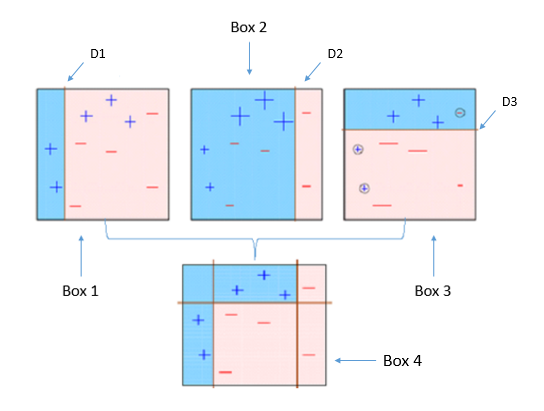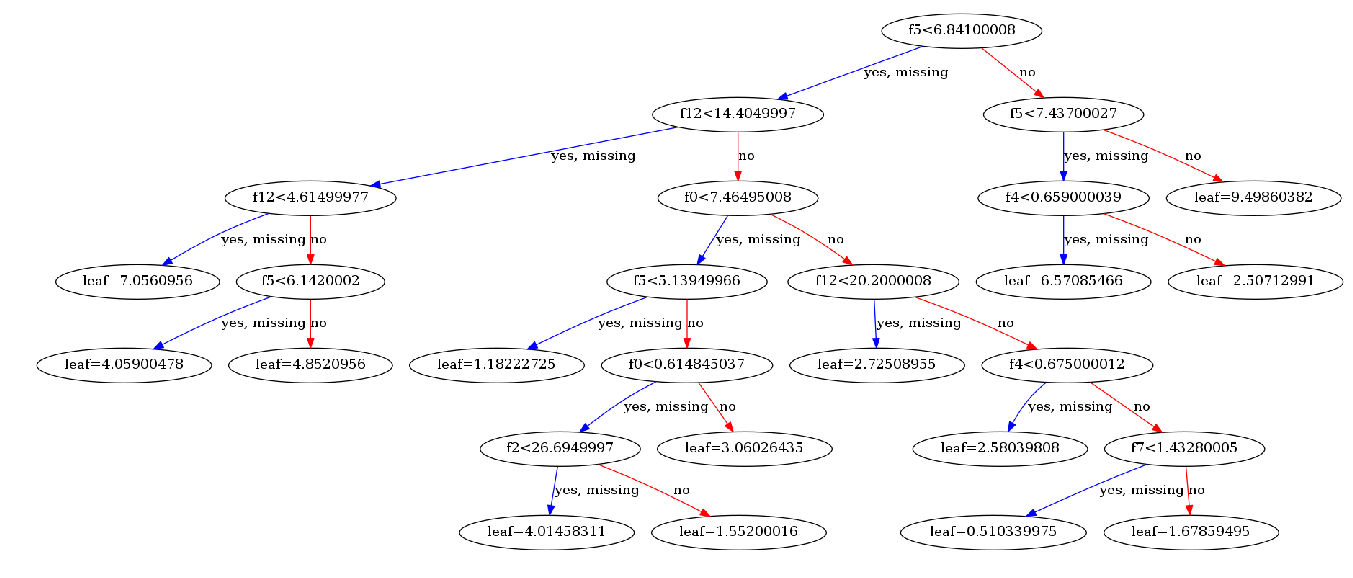## XGBoost 梯度提升算法入门

4467 字 · 2588 阅读 · 2020 年 03 月 10 日XGBoost 最早的雏形出现在 2014 年，当时由 陈天奇 读博期间负责的研究项目中。后经开源，逐渐发展成一个支持 C++，Java，Python，R 和 Julia 语言的成熟框架。XGBoost 是 Extreme Gradient Boosting 的缩写，其中的 Gradient Boosting 实际上就是梯度提升算法。所以在正式学习之前，我们有必要对梯度提升算法进行简单了解。

### 梯度提升算法import numpy as np
from sklearn.tree import DecisionTreeRegressor

# 建议一个最大为 1 层的回归树弱学习器
weak_learner = DecisionTreeRegressor(max_depth=1)

x = np.array([, [-2], [-1], , ])  # 输入值
y = np.array([-1, 13, 5, 2, 9])  # 真实值

weak_learner.fit(x, y)  # F(x) 回归树

y_ = weak_learner.predict(x)  # 预测
y_

    array([ 3.75, 13.  ,  3.75,  3.75,  3.75])

$$\mathrm{MSE}=\frac{1}{n} \sum_{i=1}^{n}\left(y_{i}-F(x_{i})\right)^{2}$$

# 计算 MSE 值
np.square(np.subtract(y, y_)).mean()

    10.95

res = y - y_
res

    array([-4.75,  0.  ,  1.25, -1.75,  5.25])

weak_learner.fit(x, res)  # h(x)

res_ = weak_learner.predict(x)
res_

    array([-1.3125, -1.3125, -1.3125, -1.3125,  5.25  ])

res - res_

    array([-3.4375,  1.3125,  2.5625, -0.4375,  0.    ])

# 计算 MSE 值
np.square(np.subtract(res, res_)).mean()

    4.059375

• 先利用弱学习器训练 $F(x)$。
• 再利用 $F(x)$ 的残差作为目标，训练 $h(x)$。
• 利用 $F(x)+h(x)$ 的残差作为目标，训练出 $m(x)$，以此类推。
• 最后 $F(x)+h(x)+m(x)+…$ 就是最终的强学习器模型。

$$\mathrm{L(y, F(x))}=\frac{1}{n} \sum \left(y-F(x)\right)^{2}$$

$$\frac{\partial L}{\partial F}=- \frac{2}{n} \sum (y-F)$$

$$h(x) \approx y−F(x) = - \frac{n}{2} \frac{\partial L}{\partial F} = - \lambda \frac{\partial L}{\partial F}$$

$$H(x) = F(x) - \lambda \frac{\partial L}{\partial F}$$

### XGBoost 框架使用

#### 分类问题

XGBoost 使用起来非常方便，高效。如果你之前有使用 scikit-learn 的经验，上手该工具简直不要太简单。接下来，我们将通过几个 Toy Dataset 来学习 XGBoost 的使用技巧。首先，我们选择一个简单的分类问题：手写字符识别。同样，实验选择 scikit-learn 提供的相关函数和方法，如果你不太熟悉 scikit-learn 的使用，可以先学习 scikit-learn 机器学习基础课程

from sklearn.datasets import load_digits

X = digits.data  # 特征值
y = digits.target  # 目标值

X.shape, y.shape

    ((1797, 64), (1797,))

from sklearn.model_selection import train_test_split

# 划分数据集，70% 训练数据和 30% 测试数据
X_train, X_test, y_train, y_test = train_test_split(X, y, test_size=0.3)

X_train.shape, X_test.shape, y_train.shape, y_test.shape

    ((1257, 64), (540, 64), (1257,), (540,))

• max_depth – 基学习器的最大树深度。
• learning_rate – Boosting 学习率。
• n_estimators – 决策树的数量。
• gamma – 惩罚项系数，指定节点分裂所需的最小损失函数下降值。
• booster – 指定提升算法：gbtree, gblinear or dart。
• n_jobs – 指定多线程数量。
• reg_alpha – L1 正则权重。
• reg_lambda – L2 正则权重。
• scale_pos_weight – 正负权重平衡。
• random_state – 随机数种子。

import xgboost as xgb

model_c = xgb.XGBClassifier()
model_c

    XGBClassifier(base_score=None, booster=None, colsample_bylevel=None,
colsample_bynode=None, colsample_bytree=None, gamma=None,
gpu_id=None, importance_type='gain', interaction_constraints=None,
learning_rate=None, max_delta_step=None, max_depth=None,
min_child_weight=None, missing=nan, monotone_constraints=None,
n_estimators=100, n_jobs=None, num_parallel_tree=None,
objective='binary:logistic', random_state=None, reg_alpha=None,
reg_lambda=None, scale_pos_weight=None, subsample=None,
tree_method=None, validate_parameters=False, verbosity=None)

model_c.fit(X_train, y_train)  # 使用训练数据训练
model_c.score(X_test, y_test)  # 使用测试数据计算准确度

    0.9518518518518518

#### 回归问题

from sklearn.datasets import load_boston

X = boston.data  # 特征值
y = boston.target  # 目标值

# 划分数据集，80% 训练数据和 20% 测试数据
X_train, X_test, y_train, y_test = train_test_split(X, y, test_size=0.2)

X_train.shape, X_test.shape, y_train.shape, y_test.shape

    ((404, 13), (102, 13), (404,), (102,))

model_r = xgb.XGBRegressor()
model_r

    XGBRegressor(base_score=None, booster=None, colsample_bylevel=None,
colsample_bynode=None, colsample_bytree=None, gamma=None,
gpu_id=None, importance_type='gain', interaction_constraints=None,
learning_rate=None, max_delta_step=None, max_depth=None,
min_child_weight=None, missing=nan, monotone_constraints=None,
n_estimators=100, n_jobs=None, num_parallel_tree=None,
objective='reg:squarederror', random_state=None, reg_alpha=None,
reg_lambda=None, scale_pos_weight=None, subsample=None,
tree_method=None, validate_parameters=False, verbosity=None)
model_r.fit(X_train, y_train)  # 使用训练数据训练
model_r.score(X_test, y_test)  # 使用测试数据计算 R^2 评估指标

    0.7859600854280273

#### 目标参数

model_c.get_params()

    {'objective': 'multi:softprob',
'base_score': 0.5,
'booster': None,
'colsample_bylevel': 1,
'colsample_bynode': 1,
'colsample_bytree': 1,
'gamma': 0,
'gpu_id': -1,
'importance_type': 'gain',
'interaction_constraints': None,
'learning_rate': 0.300000012,
'max_delta_step': 0,
'max_depth': 6,
'min_child_weight': 1,
'missing': nan,
'monotone_constraints': None,
'n_estimators': 100,
'n_jobs': 0,
'num_parallel_tree': 1,
'random_state': 0,
'reg_alpha': 0,
'reg_lambda': 1,
'scale_pos_weight': None,
'subsample': 1,
'tree_method': None,
'validate_parameters': False,
'verbosity': None}

#### 原生接口

DMatrix 是一种可以同时将特征和目标值封装在一起的数据类型。该方法使用简单，不再单独罗列参数，直接演示。

# 依次传入特征和目标值
train_d = xgb.DMatrix(data=X_train, label=y_train)
test_d = xgb.DMatrix(data=X_test, label=y_test)
train_d, test_d

    (<xgboost.core.DMatrix at 0x7fd8029eb390>,
<xgboost.core.DMatrix at 0x7fd8029eb358>)

XGBoost 既然想让你把特征和目标封装在一起，当然是有自己的考虑。所以，XGBoost 还提供了一个原生的训练接口 XGBoost.train。该方法的使用和上面不太一样，我们可以直接传入 DMatrix 数据，而不需要像 scikit-learn 那样分别传入特征值和目标值。

XGBoost.train 使用时，必须传入 paramsdtrain，即学习器配置和训练数据。params 至少需要传入学习目标参数。

model_t = xgb.train(params={'objective': 'reg:squarederror'}, dtrain=train_d)
model_t

    <xgboost.core.Booster at 0x7fd8029eb748>

model_t.eval(test_d)  # 使用测试数据进行评估

    '\teval-rmse:4.958941'

#### 绘制决策树

XGBoost 提供了 xgb.plot_tree 方法，可以将模型训练好之后的决策子树绘制出来。使用时，只需要传入模型和子树的序号即可，想画哪颗就画哪颗。

from matplotlib import pyplot as plt
from matplotlib.pylab import rcParams
%matplotlib inline

# 设置图像大小
rcParams['figure.figsize'] = [50, 10]

xgb.plot_tree(model_t, num_trees=1)#### 交叉验证

# 依次传入特征和目标值
boston_d = xgb.DMatrix(data=X, label=y)
xgb.cv(dtrain=boston_d, params={'objective': 'reg:squarederror'}, nfold=5, as_pandas=True)

train-rmse-mean train-rmse-std test-rmse-mean test-rmse-std
0 17.105578 0.129116 17.163215 0.584297
1 12.337973 0.097558 12.519736 0.473458
2 8.994071 0.065756 9.404534 0.472309
3 6.629481 0.050323 7.250335 0.500342
4 4.954406 0.033209 5.920812 0.591874
5 3.781454 0.029604 5.045190 0.687971
6 2.947767 0.038786 4.472030 0.686492
7 2.357748 0.042040 4.179314 0.737935
8 1.951907 0.044972 3.979878 0.798198
9 1.660895 0.044894 3.870751 0.812331

#### 在线学习•